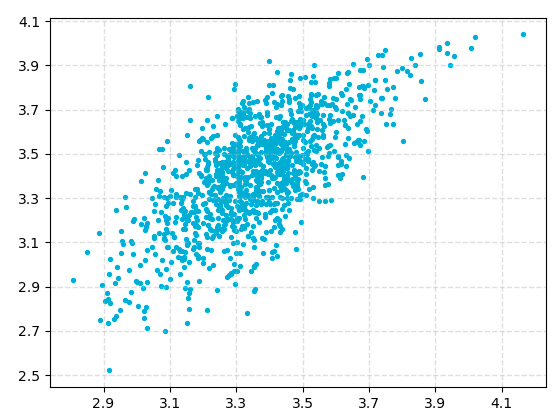# AccuracyPREDICTED VALUES

## The correlation between actual and predicted values is 0.748!! The mean absolute error is 0.133!!

This is a graph of the correlation coefficient between actual values and predicted values in Package Design Preference Score AI.
The correlation coefficient is a value between -1 and 1, and the closer it is to 1, the more accurate it is.

The mean absolute error is the average of the absolute error between the actual value and the predicted value.
Since the maximum of preference score is 5, the average error is about 3%.

Correlation chart between actual and predicted values